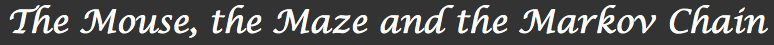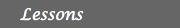1. Introduction2. The mouse and the maze 3. Processes, matrices and Markov chains 4. Will the mouse ever find the exit? How fast? 5. Other examples and applicationsIntroduction

The Math Explorer’s Club is an NSF supported project aiming at developing materials and activites to give middle school and high school students an experience of more advanced topics in mathematics.

In this activity, we introduce and develop the notion of Markov chains, consolidating the student’s grasp of (simple) probability theory and introducing two important discrete mathematics concepts: the relationship between graphs and matrices, and recurrence relations.

The material developed here is not difficult and with graphing calculators, the computations are not hard. A basic understanding of probability theory is assumed though. The reader might want to consider having a look at the Probability math explorer’s write-up, for example.

We first introduce the example of a mouse in a maze and develop the idea of a transition probability. After playing with this toy model, the next section introduces the framework of Markov chains and their matrices showing how it makes it easier to deal with problems like that of the mouse in great generality. We then investigate the model and then provide a few applications to practice the learned skills.